# Deep Dive On Regression And Classification In Machine Learning

0
230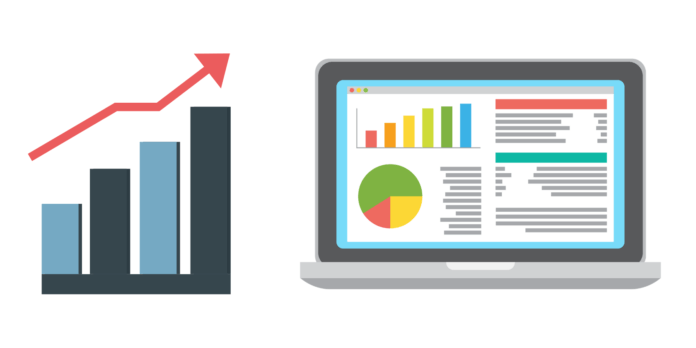Classification and regression are two of the most common prediction problems in data mining and machine learning. Fundamentally, classification and regression are both about predicting a label and a quantity.

Regression analysis is a type of predictive modeling approach that examines the relationship between two variables. Regression analysis can evaluate the strength of predictors or the influence of independent variables on dependent variables. It may also be utilized for trend forecasting, which is the ability to predict the outcome of certain events by analyzing the past patterns between variables.

Logistic regression, linear regression, polynomial regression, lasso regression, and ridge regression are some of the many forms of regression analysis.

The process of classifying a set of data is known as classification. It works with both structured and unstructured data. The first step in the procedure is predicting the class of supplied data points. The classes are often referred to as targets, labels, or categories. Predictive classification modeling is the task of estimating the mapping functions from input variables to discrete output variables. In a nutshell, the primary goal of classification is to classify data into several classes or labels to which it belongs.

Classification is of three types – Binary classification, Multi-class classification, and Multi-label classification. Binary classification has only two outcomes, such as true or false. Multi-class classification refers to classification that has more than two classes. Each sample is associated with only one label or target in multi-class classification. Multi-label classification is a type of classification in which each sample is allocated to a collection of labels or objectives.

Many classification algorithms exist, including Decision tree, Naive Bayes, Random forests, Stochastic gradient descent, and so on.

This article will cover linear regression, logistic regression, binary classification, and multi-class classification with their examples in the Python programming language.

## Linear Regression

Linear regression is a statistical technique for modeling the relationship between a dependent variable and a collection of independent variables. It plots a best-fit straight line, commonly known as the line of regression, to discover the relationship between the independent and dependent variables. It converts a continuous variable ‘x’ into a continuous variable ‘y’. It calculates the weighted sum of input characteristics and a constant called bias to make the predictions. The mathematical representation of the line of regression is y = mx + c. Here, ‘m’ represents the slope of the regression line, and ‘c’ is the bias constant.

### Example of Linear Regression in Python

For this demo, we will be using the dataset named “Students Performance in Exams” downloaded from Kaggle.

This dataset contains the following attributes :

1. Gender
2. Race/Ethnicity
3. Parental level of education
4. Lunch
5. Test preparation course
6. Maths score
8. Writing score

Numpy, Pandas, Matplotlib, and Sklearn are the external libraries required. The Numpy library is utilized for mathematical operations, while the Pandas library is used for reading the dataset, and the Sklearn library is used to train the regression model.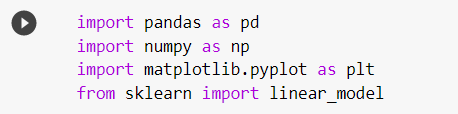We read the training and testing datasets using the Pandas library to read the .csv files and plot the training dataset on a scatter plot.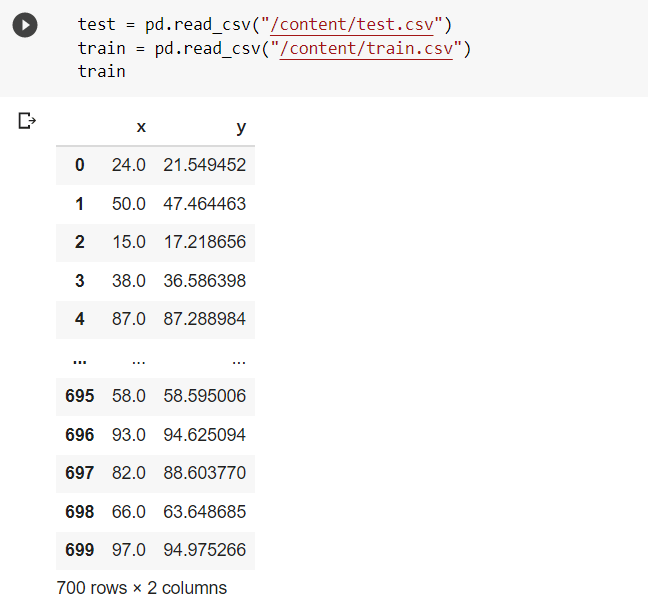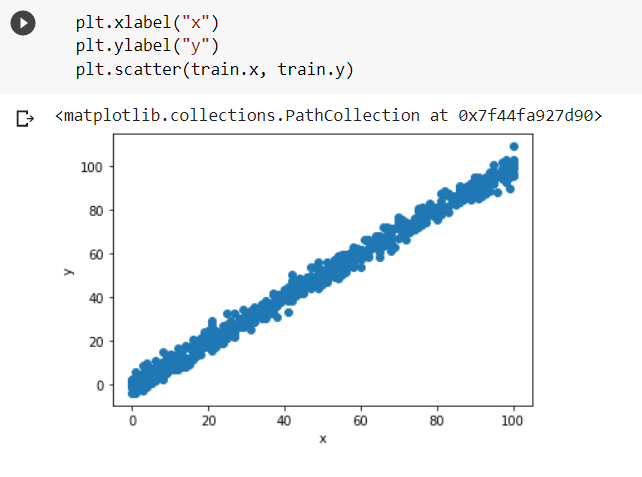Let us now use the fit() function from the sklearn package to train the regression model.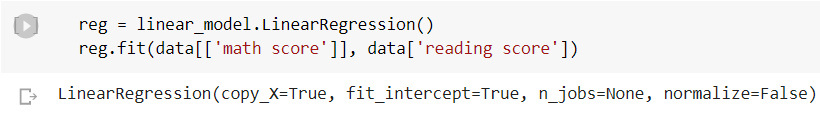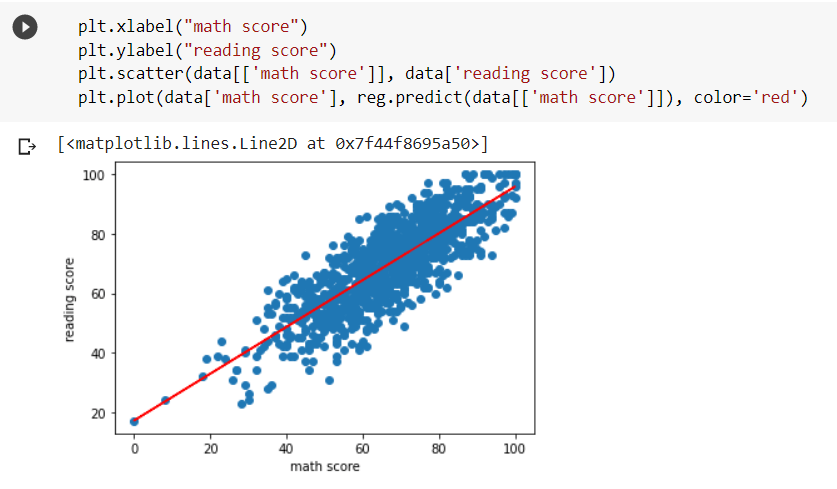Here we can see the visual representation of the line of regression on the scatter plot. The red line is the best-fit line for predicting the ‘reading score’ based on the ‘math score’.

### Logistic Regression

Logistic regression is a supervised classification algorithm. For a given collection of features (x), the target variable (y) can only take discrete values in a classification problem. Logistic regression models the data using the sigmoid function, much like linear regression assumes that the data follow a linear distribution. Thus, it produces results in a binary format which is used to predict the outcome of a categorical dependent variable. So the output is discrete (or binary like 0 or 1, yes or no, true or false, etc.).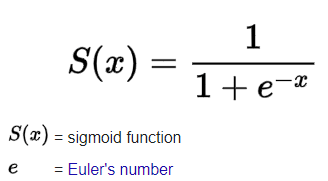An application of Logistic Regression is shown in the next section for implementing a Binary Classification model in Python.

## Binary Classification

Binary classification classifies the input variables into two binary groups (usually ‘True’ or ‘False’). Some famous use cases of binary classification include e-mail spam detection (whether an email is spam or not spam), customer behavior prediction (whether a customer will buy a product or not), etc. A binary classification model can be implemented using popular algorithms like Logistic Regression, Decision Tree, Naive Bayes, Support Vector Machine, or K-Nearest Neighbours.

### Example of Binary Classification using Logistic Regression in Python

We will use the Logistic Regression algorithm to construct a binary classification model. To train the model, we build a data frame called ‘df’ with two columns: “Score”, which reflects the grade of students, and “Test Course Completed”, which indicates whether or not the student has completed the test course. ‘1’ indicates the student has completed the test course, and ‘0’ indicates that the student has not completed the test course. Also, a similar data frame named test_df is created for testing the model.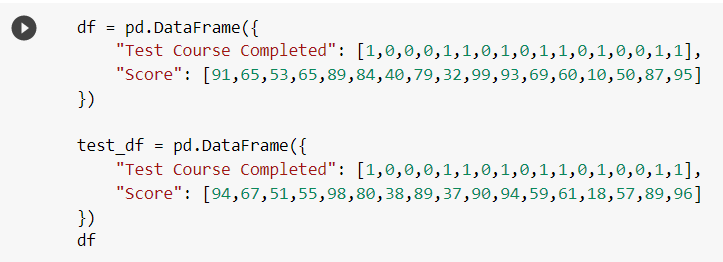The following code snippet shows the scatter plot of the training dataset.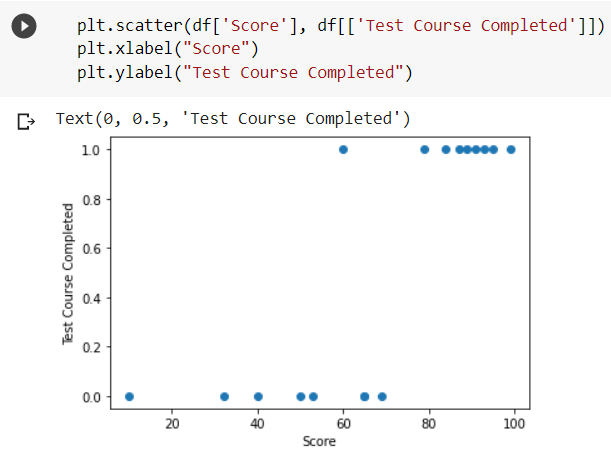The model is now ready to be trained with the help of the sklearn fit() method of the Scikit-learn library.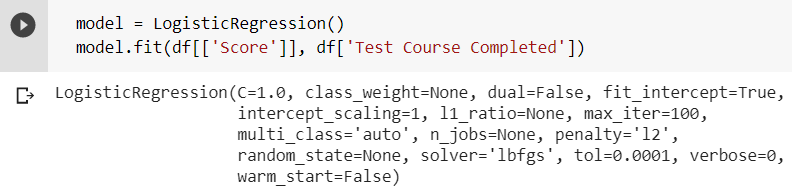The model is now ready to make predictions after it has been trained. We create a heatmap of the confusion matrix of the predicted variable, which will aid in evaluating the model more conveniently. The following code snippet depicts the confusion matrix.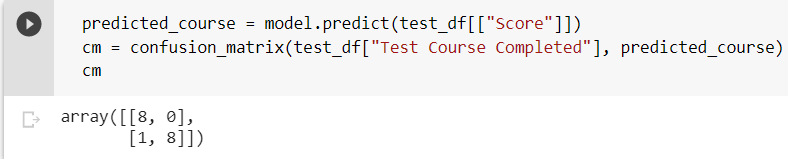Plotting this matrix on a heatmap shows the accuracy of the model.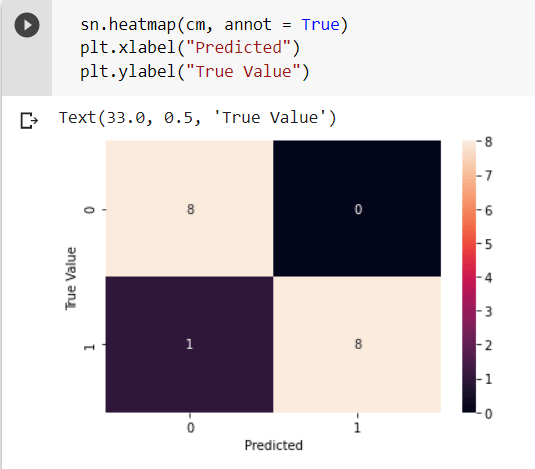As observed from the heatmap, the model has made a wrong prediction only once, implying it has considerably good accuracy. Hence, the output is classified into two groups – whether the student has completed the test course or not completed test course.

### Multi-Class Classification

In Multi-class Classification, the variables are classified into two or more discrete classes or labels. Some famous use cases for Multi-class classification include object classification/detection, digit recognition, etc.  The following figure illustrates the difference between binary and multi-class classification.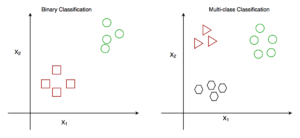### Example of Multi-Class Classification using Logistic Regression in Python

We will construct a Multi-Class Classification model for digit recognition using the logistic regression algorithm. The “Digit Dataset,” which comprises 1797 8×8 pictures of handwritten digits, is used to train and test the model.

The external libraries required are matplotlib for graphical representation and sklearn to train the model, load the dataset, and split the dataset.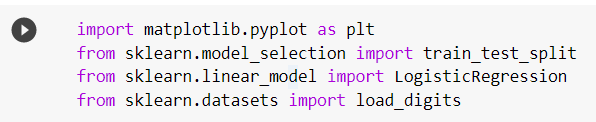The following code snippet loads the dataset and displays the attributes present in the dataset.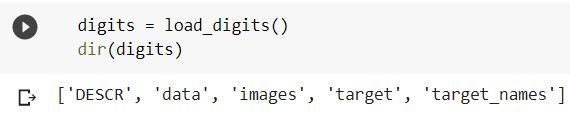The names of the digits are present in the ‘target’ variable. A sample image from the dataset is displayed below.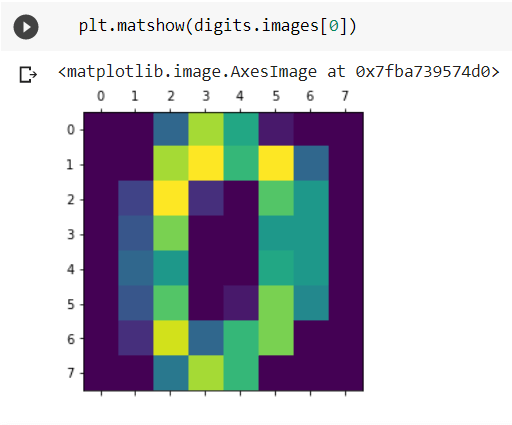Now, the dataset is split into training and testing datasets. The training dataset contains 80% of the data, and the test dataset includes 20% of the total data.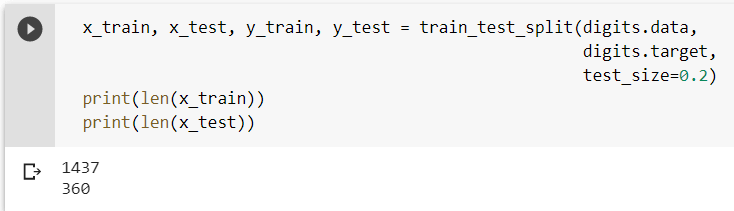Now, the model is trained using the training dataset with the help of the fit() method.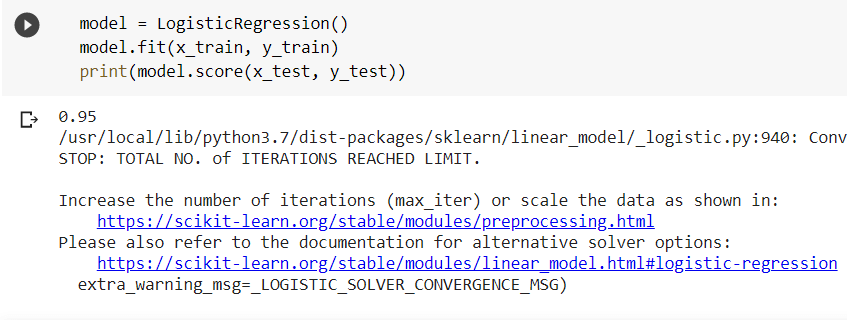The model has pretty good accuracy and can predict digits with about 95% accuracy. This can be tested by predicting a few digits from the testing dataset, as shown in the following code snippet.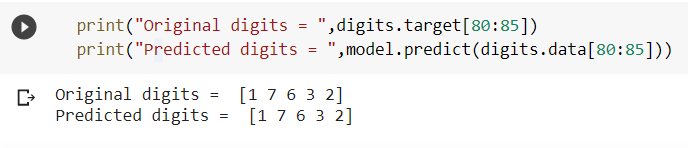The model can be evaluated, more precisely, by plotting the confusion matrix in the form of a heatmap.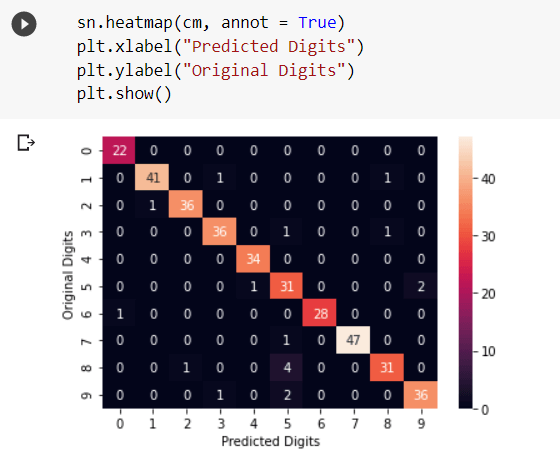The heatmap can be interpreted as 22 times the original value was ‘0’ and the model predicted them as ‘0’, but once it predicted an image of ‘0’ as ‘6’ and so on.

## Conclusion

To conclude, Regression analysis and Classification are great tools for constructing predictive and classification models in machine learning. The regression algorithms can be conveniently used in models used for predicting future outcomes of certain events and also in classification techniques that classify the data into their respective classes by accurately analyzing the relation between the variables or features. We have gone through a few regression and classification techniques in this article. Some of the more sophisticated regression and classification algorithms and techniques which can be explored include Naive Bayes Algorithm, Lasso Regression, Ridge Regression, Kernel SVM, Decision Tree Classification, Random Forest Classification, K-Nearest Neighbours, and Support Vector Machines.

Also Read: DataOps: A Product of the Machine Learning Era